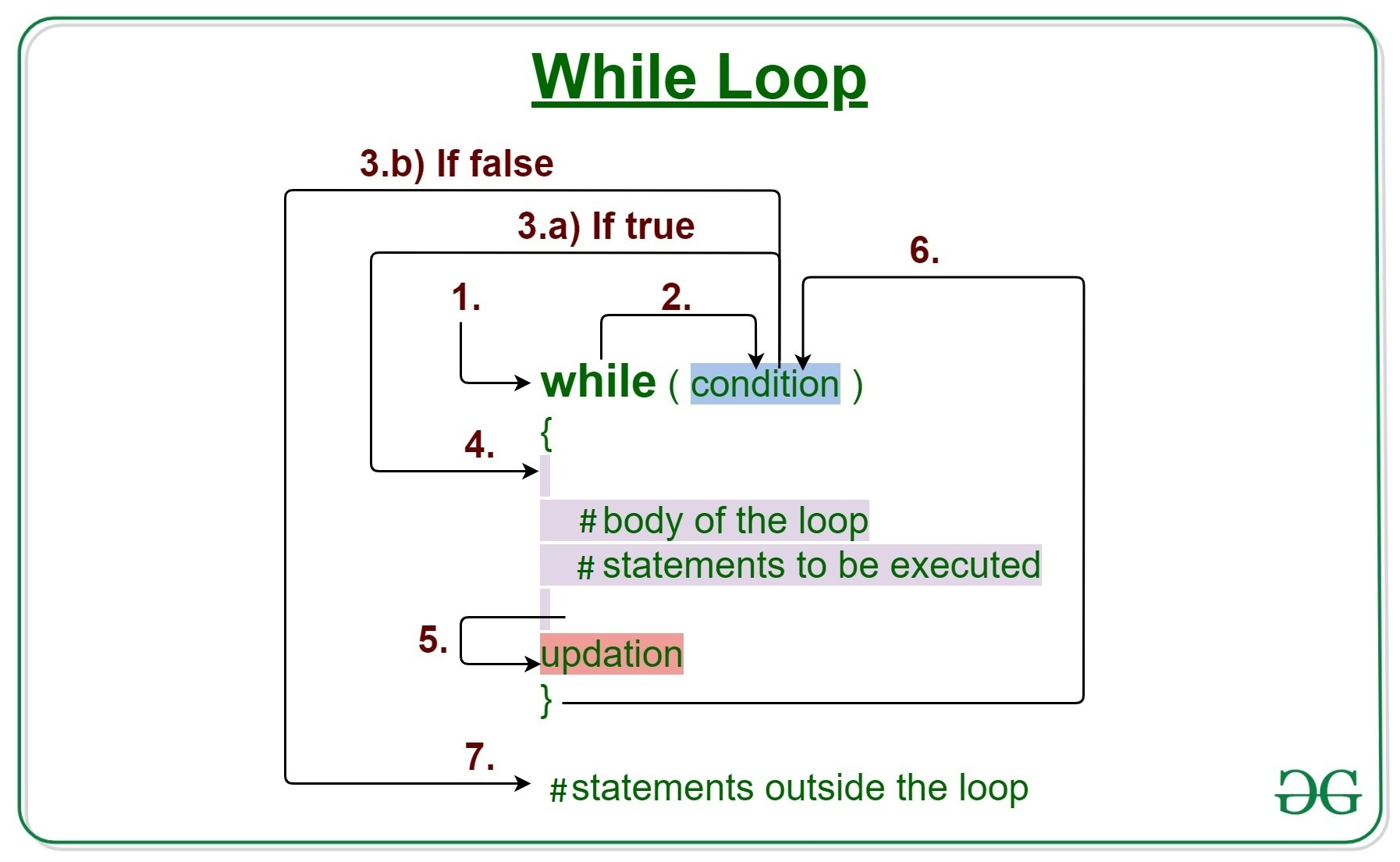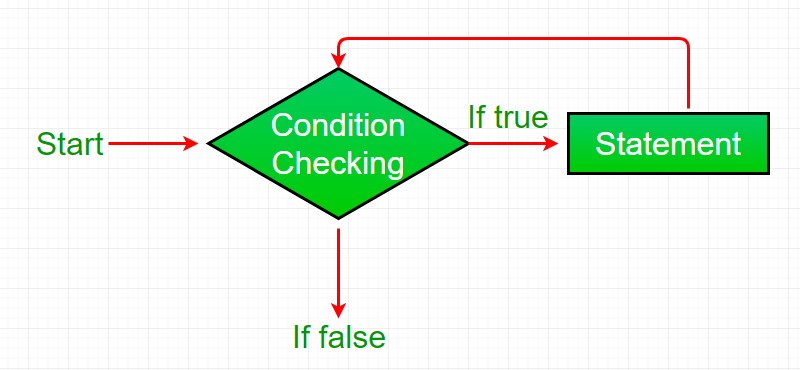Open In App
Related Articles
• Write an Interview Experience
• R Language Tutorial

# R – while loop

While loop in R programming language is used when the exact number of iterations of a loop is not known beforehand. It executes the same code again and again until a stop condition is met. While loop checks for the condition to be true or false n+1 times rather than n times. This is because the while loop checks for the condition before entering the body of the loop.

### R- While loop Syntax:

```while (test_expression) {
statement
update_expression
}  ```## How does a While loop execute?

• Control falls into the while loop.
• The flow jumps to Condition
• Condition is tested.
• If the Condition yields true, the flow goes into the Body.
• If the Condition yields false, the flow goes outside the loop
• The statements inside the body of the loop get executed.
• Updation takes place.
• Control flows back to Step 2.
• The while loop has ended and the flow has gone outside.

## Important Points about while loop in R language:

• It seems to be that while the loop will run forever but it is not true, condition is provided to stop it.
• When the condition is tested and the result is false then the loop is terminated.
• And when the tested result is True, then the loop will continue its execution.

## R – while loop Flowchart:R – while loop

Example 1:

## R

 `# R program to illustrate while loop` `result <- ``c``(``"Hello World"``)``i <- 1` `# test expression``while ``(i < 6) {` `   ``print``(result)``   ` `   ``# update expression``   ``i = i + 1``}`

Output:

``` "Hello World"
 "Hello World"
 "Hello World"
 "Hello World"
 "Hello World"```

Example 2:

## R

 `# R program to illustrate while loop` `result <- 1``i <- 1` `# test expression``while ``(i < 6) {` `   ``print``(result)``   ` `   ``# update expression``   ``i = i + 1``   ``result = result + 1``}`

Output:

``` 1
 2
 3
 4
 5```

## R – while loop break

Here we will use the break statement in the R programming language. The break statement in R is used to bring the control out of the loop when some external condition is triggered.

## R

 `# R program to illustrate while loop` `result <- ``c``(``"Hello World"``)``i <- 1` `# test expression``while ``(i < 6) {` `    ``print``(result)``    ` `    ``if``( i == 3){``        ``break``}``    ``# update expression``    ``i = i + 1``}`

Output:

``` "Hello World"
 "Hello World"
 "Hello World"```

## R

 `# Set an initial value for a variable``x <- 1` `# Loop while x is less than 10``while ``(x < 10) {``  ``if ``(x == 3) {``    ``# Skip iteration when x is 3``    ``x <- x + 1``    ``next``  ``}``  ``print``(``paste``(``"The current value of x is:"``, x))``  ``x <- x + 1``}` `# Print a message after the loop has finished``print``(``"The loop has ended."``)`

Output

``` "The current value of x is: 1"
 "The current value of x is: 2"
 "The current value of x is: 4"
 "The current value of x is: 5"
 "The current value of x is: 6"
 "The current value of x is: 7"
 "The current value of x is: 8"
 "The current value of x is: 9"```

In this instance, x’s initial value is set to 1 at the beginning. Then, as long as x is less than 10, we continue iterating using a while-loop. We use an if statement inside the loop to see if x equals 3. If so, the loop’s current iteration is skipped in favor of the following one using the next statement. If not, we use the x – x + 1 expression to increment x by 1 and output a message stating the current value of x.

The next line instructs R to move on to the next iteration of the loop and skip the current one. based on a condition, over a subset of the loop’s iterations without ever leaving the loop.

## R

 `x <- 1` `while ``(x <= 10) {``  ``if ``(x %% 2 == 0) {``    ``print``(``paste``(x, ``"is even"``))``  ``} ``else` `{``    ``print``(``paste``(x, ``"is odd"``))``  ``}``  ``x <- x + 1``}`

Output

``` "1 is odd"
 "2 is even"
 "3 is odd"
 "4 is even"
 "5 is odd"
 "6 is even"
 "7 is odd"
 "8 is even"
 "9 is odd"
 "10 is even"```

In this illustration, we initialize a variable x to 1, and then we iterate through the integers 1 through 10 using a while loop. We utilize an if-else statement inside the loop to determine whether x is even or odd. We publish a message stating that x is even if it is. We print a message noting that x is unusual if it is. Then, until x is more than 10, we increase x by 1 and loop till x is greater than 10.